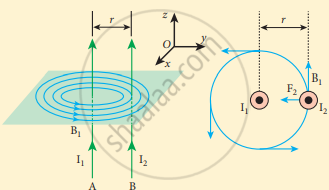Tamil Nadu Board of Secondary EducationHSC Science Class 12th

# Derive the expression for the force between two parallel, current-carrying conductors. - Physics

Numerical

Derive the expression for the force between two parallel, current-carrying conductors.

#### Solution

1. Two long parallel current-carrying conductors separated by a distance V in air.
2. I1, I2 – current passing through the conductors A and B in the same direction.
3. Magnetic field due to current I2 of elemental length dl in A
vec"B"_1 = (mu_0"I"_1)/(2pi"r") (- hat"i")
vec"B"_1 = (- mu_0"I"_1)/(2pi"r") hat"i"
4. The direction of magnetic field is perpendicular to the plane of the paper and outwards.
5. A force on a small elemental length dl in B, experience magnetic field B1.

"d"vec"F" = ("I"_2 vec"d"l xx vec"B"_1)

= - "I"_2"dl" (mu_0 "I"_1)/(2pi"r") (hat"k" xx hat"i")

"d"vec"F" = (- mu_0 "I"_1"I"_2"dl")/(2pi"r")

Force per unit length of the conductor.

vec"F"/l = (- mu_0"I"_1"I"_2)/(2pi"r") hat"j"Magnetic field due to current I2 of elemental

length dl in A

vec"B"_2 = (mu_0"I"_2)/(2pi"r") hat"i"

The direction of a magnetic field is perpendicular to the plane of the paper and outwards.

Force on conductor A is directed towards conductor B.

dF = ("I"_1 "d" vec"l" xx vec"B"_2)

= "I"_1"dl" (mu_0 "I"_2)/(2pi"r") (hat"k" xx hat"i")

"d"vec"F" = (mu_0 "I"_1"I"_2 "dl")/(2pi"r") hat"j"

Force per unit length of the conductor A,

vec"F"/l = (- mu"I"_1"I"_2)/(2pi"r") hat"j"

Attractive force – direction of electric current is same.

Repulsive force – direction of electric current is opposite.

Concept: Lorentz Force
Is there an error in this question or solution?

#### APPEARS IN

Tamil Nadu Board Samacheer Kalvi Class 12th Physics Volume 1 and 2 Answers Guide
Chapter 3 Magnetism and magnetic effects of electric current
Evaluation | Q III. 13. | Page 192
Share